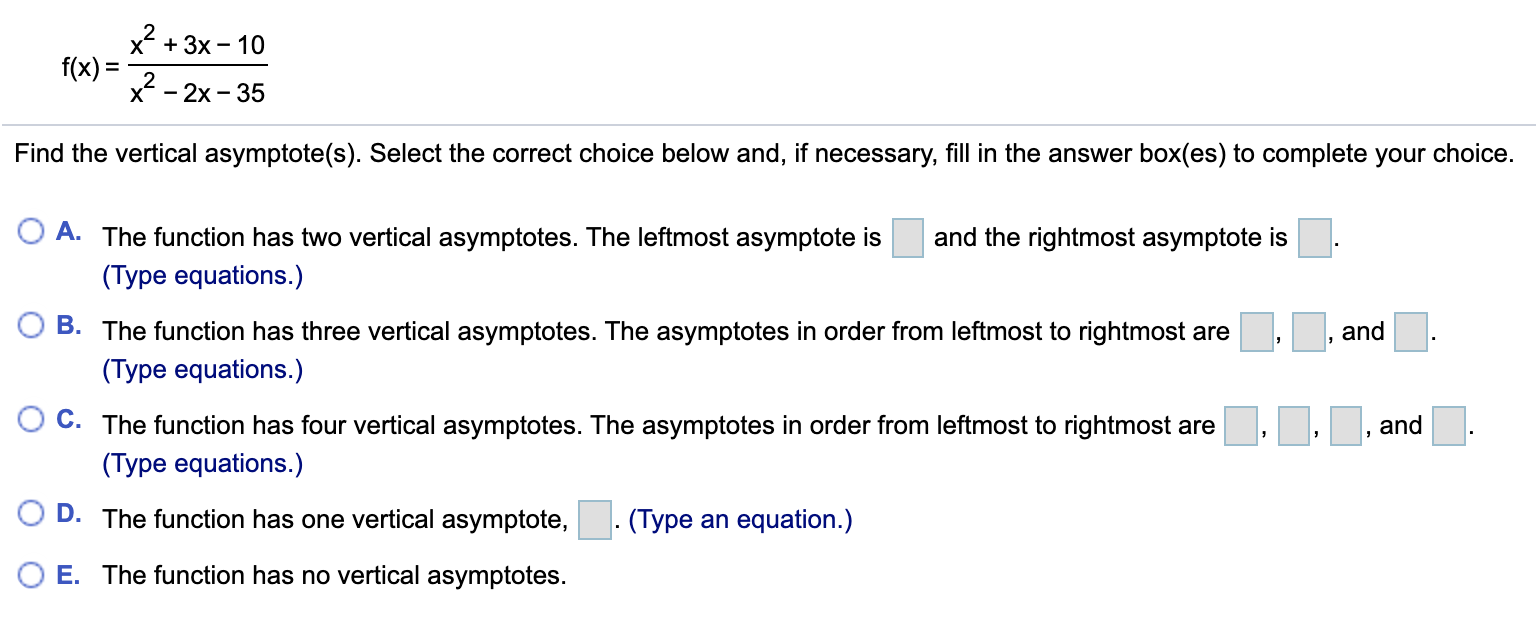# x + 3x - 10f(x) =x* - 2х- 35Find the vertical asymptote(s). Select the correct choice below and, if necessary, fill in the answer box(es) to complete your choice.O A. The function has two vertical asymptotes. The leftmost asymptote isand the rightmost asymptote is(Type equations.), andB. The function has three vertical asymptotes. The asymptotes in order from leftmost to rightmost are(Type equations.)O C. The function has four vertical asymptotes. The asymptotes in order from leftmost to rightmost areand(Type equations.)O D. The function has one vertical asymptote,. (Type an equation.)O E. The function has no vertical asymptotes.

Question
1 viewshelp_outlineImage Transcriptionclosex + 3x - 10 f(x) = x* - 2х- 35 Find the vertical asymptote(s). Select the correct choice below and, if necessary, fill in the answer box(es) to complete your choice. O A. The function has two vertical asymptotes. The leftmost asymptote is and the rightmost asymptote is (Type equations.) , and B. The function has three vertical asymptotes. The asymptotes in order from leftmost to rightmost are (Type equations.) O C. The function has four vertical asymptotes. The asymptotes in order from leftmost to rightmost are and (Type equations.) O D. The function has one vertical asymptote, . (Type an equation.) O E. The function has no vertical asymptotes. fullscreen
check_circle

Step 1

Vertical asymptotes are the vertical lines at which at denominator of the rational function is 0.

Step 2

Equate the denominator of the function f(x) to 0.

Step 3

So, left most vertical asymptote is x=-5 and rightmost ...

### Want to see the full answer?

See Solution

#### Want to see this answer and more?

Solutions are written by subject experts who are available 24/7. Questions are typically answered within 1 hour.*

See Solution
*Response times may vary by subject and question.
Tagged in

### Math